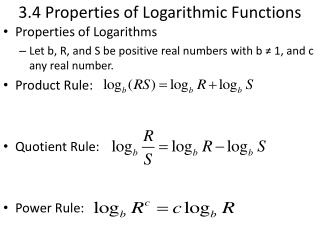DownloadDownload Presentation3.4 Properties of Logarithmic Functions

# 3.4 Properties of Logarithmic Functions

Download Presentation## 3.4 Properties of Logarithmic Functions

- - - - - - - - - - - - - - - - - - - - - - - - - - - E N D - - - - - - - - - - - - - - - - - - - - - - - - - - -
##### Presentation Transcript

1. 3.4 Properties of Logarithmic Functions • Properties of Logarithms • Let b, R, and S be positive real numbers with b ≠ 1, and c any real number. • Product Rule: • Quotient Rule: • Power Rule:

2. Expanding the Logarithm of a Product • Assuming x and y are positive, use properties of logarithms to write log (8xy4) as a sum of logarithms or multiples of logarithms.

3. Expanding the Logarithm of a Quotient • Assuming x is positive, use properties of logarithms to write as a sum or difference of logarithms or multiples of logarithms.

4. Condensing a Logarithmic Expression • Assuming x and y are positive, write ln x5 – 2 ln (xy) as a single logarithm.

5. Change of Base • When working with a logarithmic expression with an undesirable base, it is possible to change the expression into a quotient of logarithms with a different base. • Change-of-Base Formula for Logarithms: • For positive real numbers a, b, and x with a ≠ 1 and b ≠ 1,

6. Change-of-Base Formula • The following two forms are useful in evaluating logarithms and graphing logarithmic functions. OR

7. Evaluating Logarithms by Changing the Base a.) b.) c.)

8. Graphs of Logarithmic Functions with Base b • Using the change-of-base formula we can rewrite any logarithmic function g(x) = logbx as

9. Graphing Logarithmic Functions • Describe how to transform the graph of f(x) = ln x into the graph of the given function. a.) g(x) = log5x The graph is obtained by vertically shrinking the graph by a factor of

10. Graphing Logarithmic Functions • Describe how to transform the graph of f(x) = ln x into the graph of the given function. b.) h(x) = log1/4x The graph is obtained by, in any order, a reflection across the x-axis and a vertical shrink by a factor of .

11. Re-expressing Data • When seeking a model for a set of data it is often helpful to transform the data by applying a function to one or both of the variables in the data set. • Such a transformation of a data set in a re-expression of the data.

12. More Practice!!!!! • Homework – Textbook p. 317 #2 – 36 even.